Miscellaneous

Chapter 1 Class 11 Sets
Serial order wise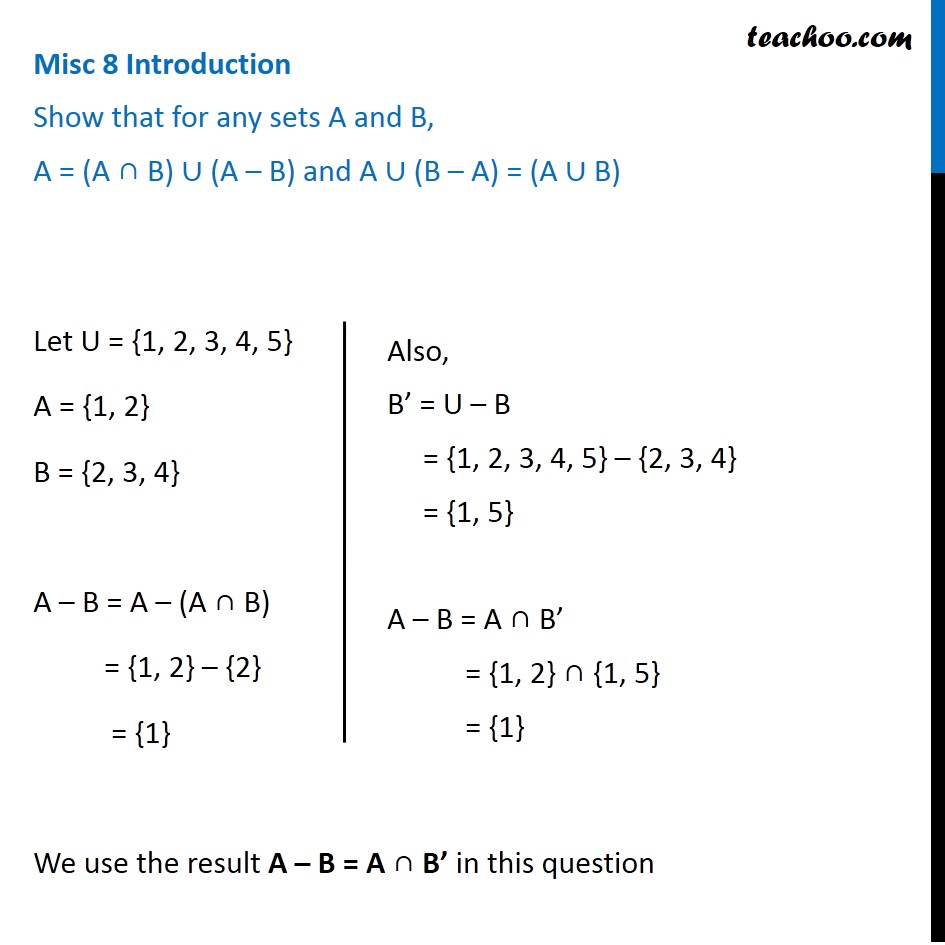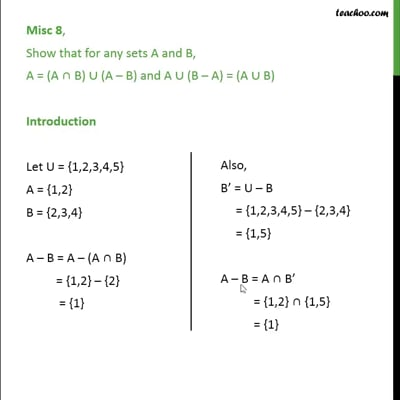This video is only available for Teachoo black users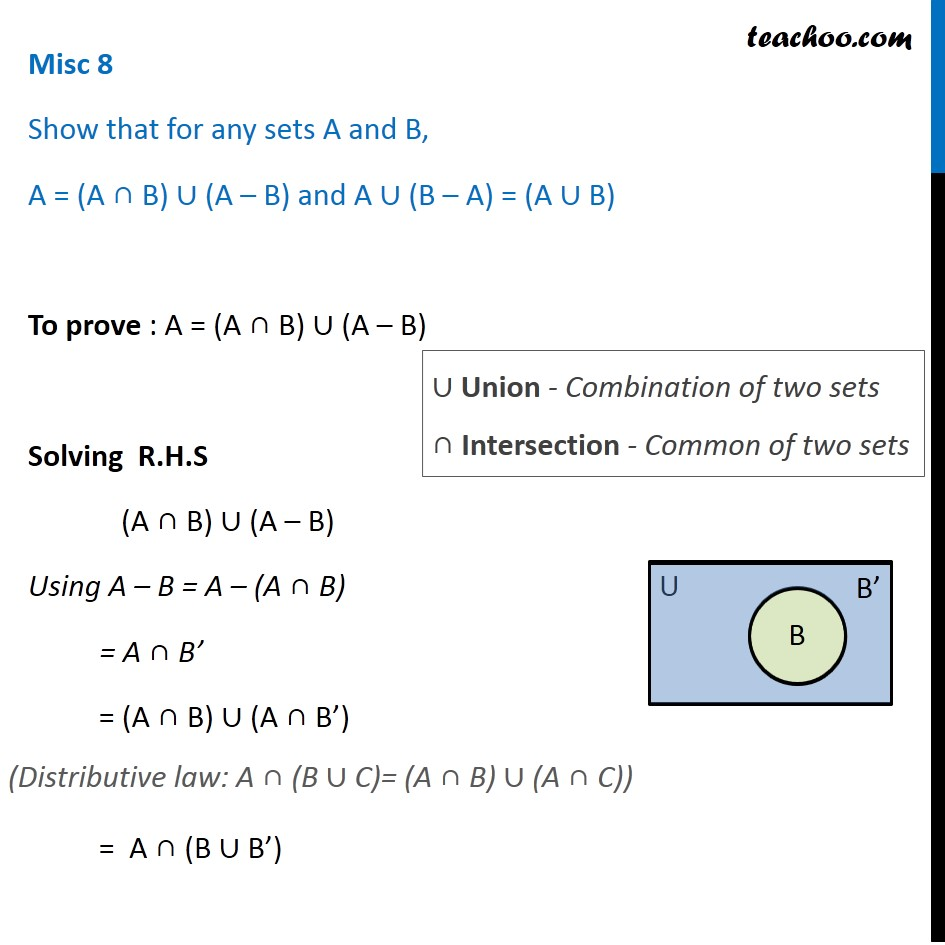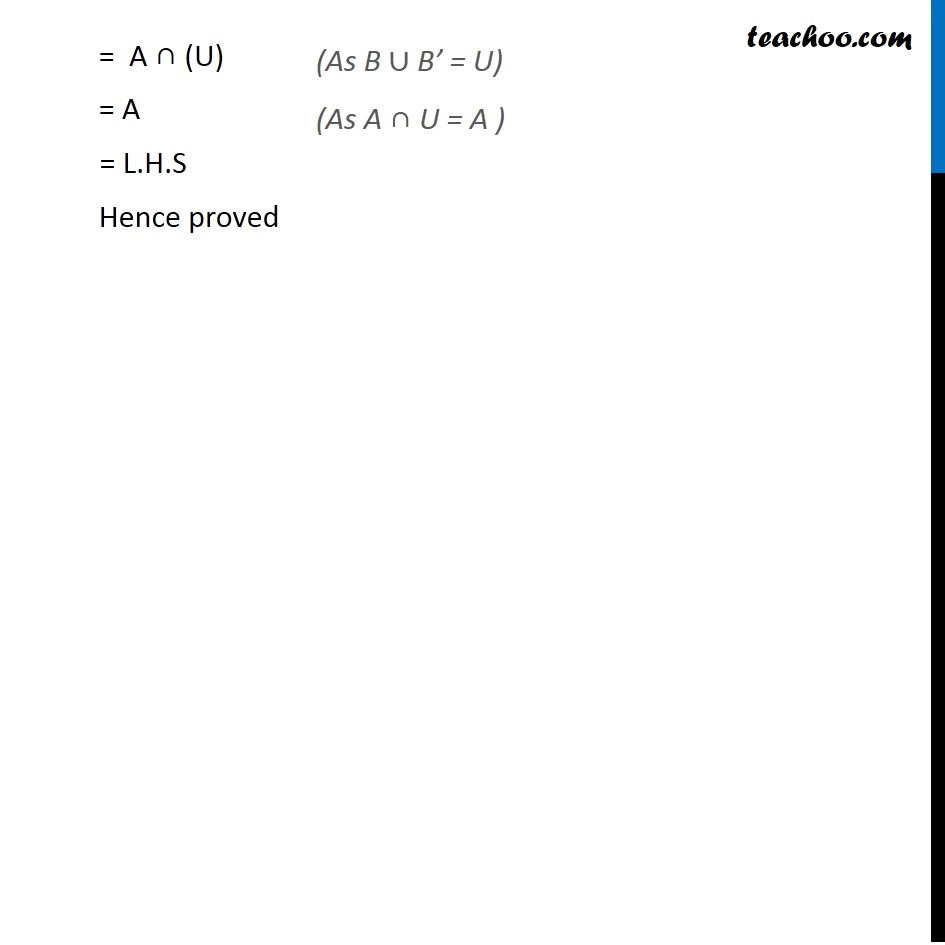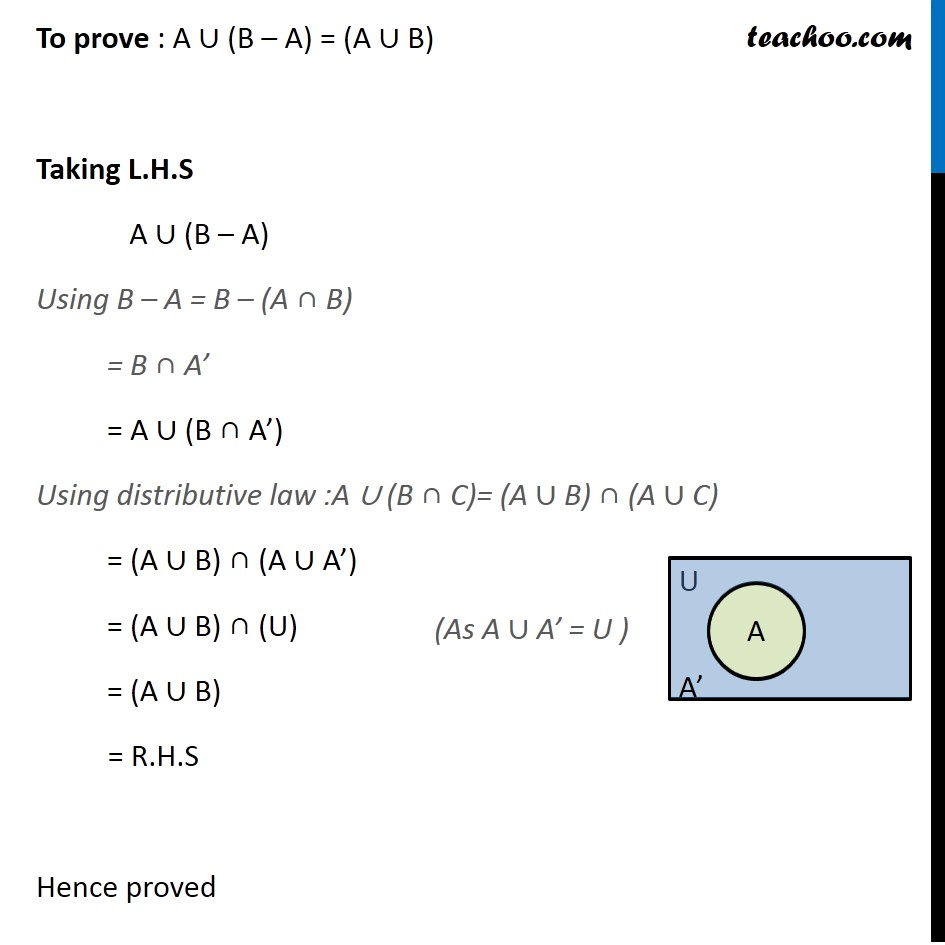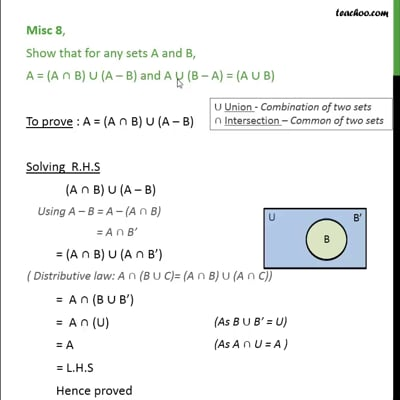This video is only available for Teachoo black users

Maths Crash Course - Live lectures + all videos + Real time Doubt solving!

### Transcript

Misc 8 Introduction Show that for any sets A and B, A = (A ∩ B) ∪ (A – B) and A ∪ (B – A) = (A ∪ B) Let U = {1, 2, 3, 4, 5} A = {1, 2} B = {2, 3, 4} A – B = A – (A ∩ B) = {1, 2} – {2} = {1} We use the result A – B = A ∩ B’ in this question Also, B’ = U – B = {1, 2, 3, 4, 5} – {2, 3, 4} = {1, 5} A – B = A ∩ B’ = {1, 2} ∩ {1, 5} = {1} Misc 8 Show that for any sets A and B, A = (A ∩ B) ∪ (A – B) and A ∪ (B – A) = (A ∪ B) To prove : A = (A ∩ B) ∪ (A – B) Solving R.H.S (A ∩ B) ∪ (A – B) Using A – B = A – (A ∩ B) = A ∩ B’ = (A ∩ B) ∪ (A ∩ B’) = A ∩ (B ∪ B’) ∪ Union - Combination of two sets ∩ Intersection - Common of two sets (Distributive law: A ∩ (B ∪ C)= (A ∩ B) ∪ (A ∩ C)) = A ∩ (U) = A = L.H.S Hence proved (As B ∪ B’ = U) (As A ∩ U = A ) To prove : A ∪ (B – A) = (A ∪ B) Taking L.H.S A ∪ (B – A) Using B – A = B – (A ∩ B) = B ∩ A’ = A ∪ (B ∩ A’) Using distributive law :A ∪ (B ∩ C)= (A ∪ B) ∩ (A ∪ C) = (A ∪ B) ∩ (A ∪ A’) = (A ∪ B) ∩ (U) = (A ∪ B) = R.H.S Hence proved (As A ∪ A’ = U )